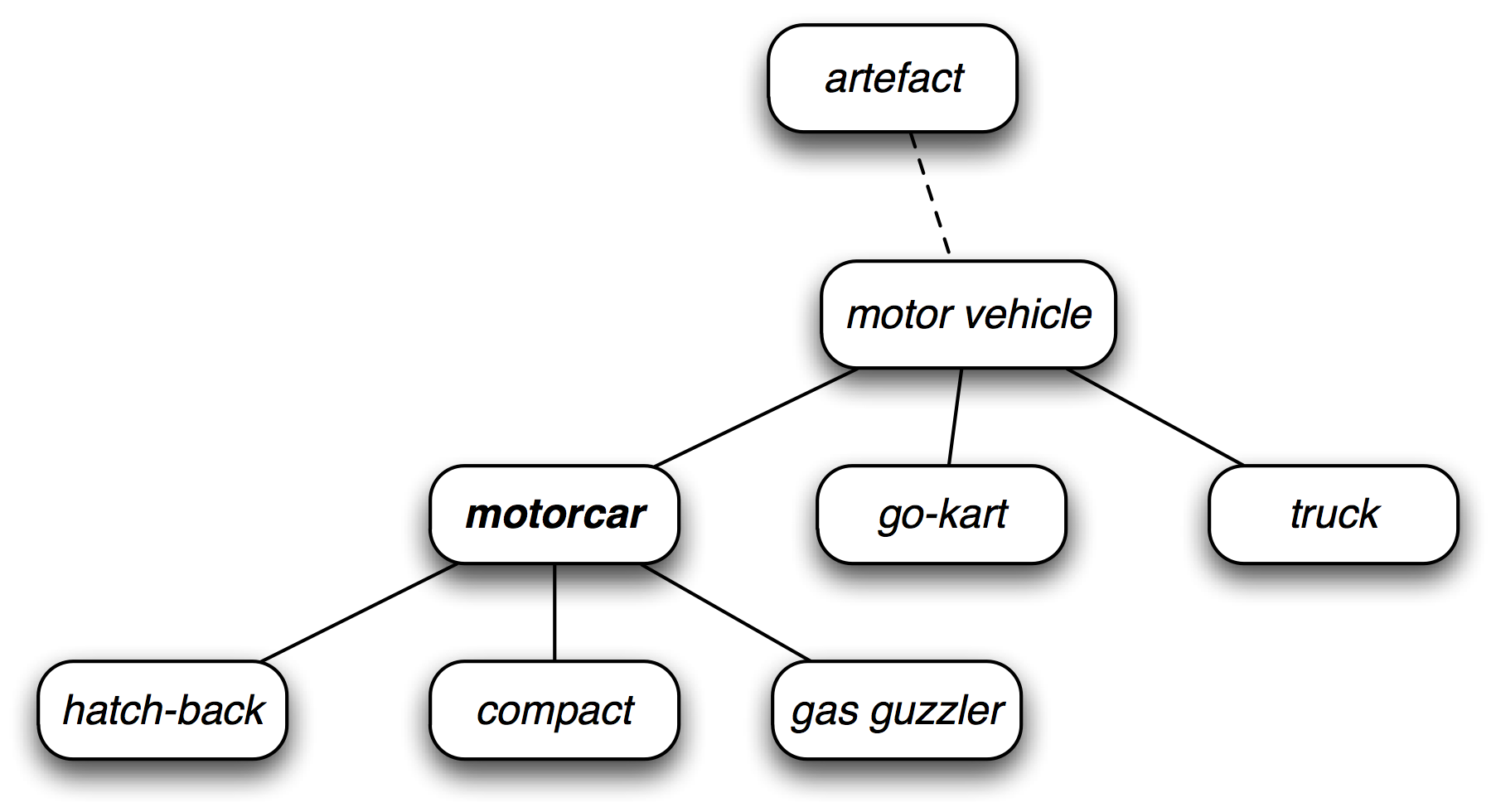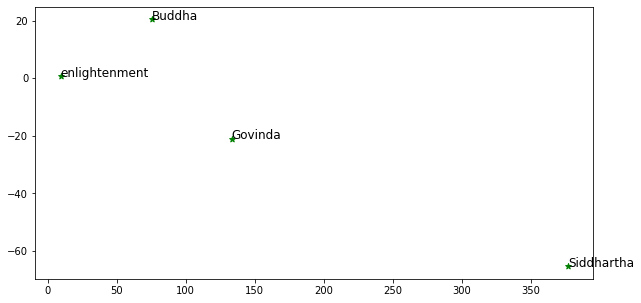Learn to Code via Tutorials on Repl.it!

← Back to all posts
##### Word vectors - Why they are fundamental for NLP and how to create them (part 1)

repl.it file

Who is this for: If you're a developer interested in NLP and don't know where to begin. Or you have a data science background and want to learn NLP.

How do you represent language in a way that can be used in NLP tasks? This means to represent words in a way that captures their meaning. For example, this is the meaning of `probability: the extent to which an event is likely to occur`.

Can you just use the default way computers represent strings? Ultimately, all data on a computer is stored as sequences of 0s and 1s. Strings are a sequence of characters and each character is represented by a number. Numbers are then internally stored in a 0/1 format. This is only useful for computers to manage words, but it doesn't capture the meaning of a word like `probability` at all.

If this was a comp sci lecture, I would be telling you about wordnets and one-hot vectors. Maybe even show you how to implement them, get you all excited, and then tell you that they don't really work well. I'll mention briefly what they are so you can understand why modern methods work better.

## WordNet

WordNet is like a thesaurus in that it groups together words based on senses of words. In the below image, you can see that `motorcar` is a motor vehicle, and it also contains subjects like `compact` and `gas guzzler`. Trying to capture relationships of each word, and all the senses of each word, is extremely difficult. Agreeing on the senses and boundaries of a word is also not simple. These are just some of the limitations of using wordnet.## One hot vectors

One method you may see in many NLP tutorials is to use one hot vectors to represent words.

``````Sentence: I have a blue dog

I:    [ 1 0 0 0 0 ]
have: [ 0 1 0 0 0 ]
a:    [ 0 0 1 0 0 ]
blue: [ 0 0 0 1 0 ]
dog:  [ 0 0 0 0 1 ]``````

First you have to create a `vocabulary`. This means a list of all the words that you will create vectors for, and that any word that is not part of your vocabulary you will represent it as an unknown word `<UNK>`. Otherwise, for words that are in the vocabulary, they are represented with a `1` that matches the index of their location in the vocabulary list, and `0` for all other words in the vocabularly.

So in the above example, we have 5 words in our vocabularly. `Blue` is in the fourth spot in our vocabularly, so it has a `1` in the 4th spot and `0` for all other words.

Although this is commonly used in beginner NLP tutorials, you can see that it doesn't capture the meaning of a word.

## Contextual meaning

In most cases, the meaning of a word is its use
Wittgenstein

Contextual meaning brings us to how modern NLP represents words. The meaning of a word is determined by how it is used. Try this thought experiment. If you didn't know what `tiktok` was, one way to find out is to gather all the sentences where `tiktok` is used. Then figure out the word by how it's used in all the sentences.

``````Example list of sentences:

Tiktok attracts these users in so many ways.
Tiktok users can shoot, edit, and share 15-second videos.

From these example sentences, you can figure out that it's a mobile app that has many users, and people use it to make and share 15-second videos. This kind of resembles how people actually learn what new words mean. But how do we create a program to do this for us?

## Co-occurrence EmbeddingsThis plot shows a co-occurrence matrix for the words Siddhartha, Govinda, Buddha, and enlightenment from the book Siddhartha by Herman Hesse. It looks like Buddha is closer to enlightenment compared to Siddhartha!

It looks interesting, but what does it actually mean? So the above plot is made using all the words from the book Siddhartha. As you go through each word, its context is the set of words that appear nearby.

``````... A goal stood before *Siddhartha*, a single goal...
... he'll find the same *Siddhartha* and Govinda ...
... and teachers, *Siddhartha* began to speak ...``````

If our window is just 1 word, then in the first sentence we see that `before` and `a` are in the context. In the second sentence, `same` and `and` are in the context. In the third sentence, `teachers` and `began` are in the context.

As a simple example, we can try to calculate the co-occurrences of this sentence: `['a', 'goal', 'stood', 'before', 'Siddhartha', 'a', 'single', 'goal']`.

*Siddharthaabeforegoalsinglestood
Siddhartha011000
a100110
before100001
goal010011
single010100
stood001100

Let's go down the left side column. The first word is `Siddhartha`, and in the sentence above we can see that there is only instance of this word. For that instance, it has two context words (one on each side): `before` and `a`. In that row, you can see there is a `1` under both of these words.

Now, let's program!

First we have to get the text from the book. Fortunately, the copyright has expired, so it's available on Project Gutenberg.

``````import urllib.request
import string

book = []
book_url_txt = 'https://www.gutenberg.org/cache/epub/2500/pg2500.txt'

# Append each line in the book to the list
for line in urllib.request.urlopen(book_url_txt):
book.append(line.decode('utf-8').strip())

# Remove the beginning opening remarks that are not useful
book = book[48:]

# Split up the sentence into individual words
book = [sent.split() for sent in book if sent != '']

# Remove punctuation from each word
book = [word.translate(str.maketrans('', '', string.punctuation))
for li in book for word in li]

# Take a look at a random set of 20 words
print(book[:20])
"""
['house', 'in', 'the', 'sunshine', 'of', 'the', 'riverbank',
'near', 'the', 'boats', 'in', 'the', 'shade', 'of', 'the',
"""``````

Now that we have our dataset, the next step is to get all the distinct words.

``````def distinct_words(corpus):
""" Determine a list of distinct words for the corpus.
Params:
corpus (list of list of strings): corpus of documents
Return:
corpus_words (list of strings): sorted list of distinct words across the corpus
num_corpus_words (integer): number of distinct words across the corpus
"""
corpus_words = []
num_corpus_words = -1

all_corpus_words = [y for x in corpus for y in x]
distinct_words = set(all_corpus_words)
corpus_words = sorted(list(distinct_words))
num_corpus_words = len(corpus_words)

return corpus_words, num_corpus_words

corpus_words, num_corpus_words = distinct_words(book)

print(num_corpus_words)
# 4470``````

We have 4470 distinct words in our book! If you `print(corpus_words)` you can see what they are. Now we're ready to create a co-occurrence matrix just like the small example above.

``````def compute_co_occurrence_matrix(corpus, window_size=4):
""" Compute co-occurrence matrix for the given corpus and window_size (default of 4).

Note: Each word in a document should be at the center of a window. Words near edges will have a smaller
number of co-occurring words.

For example, if we take the document "<START> All that glitters is not gold <END>" with window size of 4,
"All" will co-occur with "<START>", "that", "glitters", "is", and "not".

Params:
corpus (list of list of strings): corpus of documents
window_size (int): size of context window
Return:
M (a symmetric numpy matrix of shape (number of unique words in the corpus , number of unique words in the corpus)):
Co-occurence matrix of word counts.
The ordering of the words in the rows/columns should be the same as the ordering of the words given by the distinct_words function.
word2ind (dict): dictionary that maps word to index (i.e. row/column number) for matrix M.
"""
words, num_words = distinct_words(corpus)
M = None
word2ind = {}

word2ind = dict(zip(words, range(len(words))))

M = np.zeros((num_words, num_words))

# Iterate over each sentence in our book
for text in corpus:
# Iterate over each word in each sentence
for i, word in enumerate(text):
if i <= window_size:
# We don't want start_index to be less than 0
start_index = 0
else:
start_index = i - window_size
end_index = i + window_size + 1 # Need to + 1 because python slices do not include the last index number
window_words = text[start_index:end_index]
window_words.remove(word) # Don't want the center word in window_words
column_index_of_centre_word = word2ind[word] # In our sample matrix above, the left hand column contains the "centre" words
# Iterate over each context/window word
for w in window_words:
row_index_of_context_word = word2ind[w]
M[row_index_of_context_word, column_index_of_centre_word] += 1

return M, word2ind

# This function assumes that there are many corpora that are passed in.
# Since we only have one corpus (1 book), we have to insert it in a list
M, word2ind = compute_co_occurrence_matrix([book], window_size=6)

M.shape
# (4470, 4470)

print(word2ind)
"""
{
...
'anew': 700,
'anger': 701,
'angry': 702,
...
}
"""``````

The shape of this new matrix is 4470 by 4470 (number of distinct words in the book). We're almost there! How do we turn this huge matrix into something we can plot out on a 2-d graph? This means we have to turn one dimension of the matrix from 4470 down to 2. What is this magic?!

There's a technique called Singular Value Decomposition (SVD) that can do this (let me know if you want a post explaining SVD). We will use `scikit-learn`'s TruncatedSVD method which can work well with sparse matrices.

``````from sklearn.decomposition import TruncatedSVD

def reduce_to_k_dim(M, k=2):
""" Reduce a co-occurence count matrix of dimensionality (num_corpus_words, num_corpus_words)
to a matrix of dimensionality (num_corpus_words, k) using the following SVD function from Scikit-Learn:
- https://scikit-learn.org/stable/modules/generated/sklearn.decomposition.TruncatedSVD.html

Params:
M (numpy matrix of shape (number of unique words in the corpus , number of unique words in the corpus)): co-occurence matrix of word counts
k (int): embedding size of each word after dimension reduction
Return:
M_reduced (numpy matrix of shape (number of corpus words, k)): matrix of k-dimensioal word embeddings.
In terms of the SVD from math class, this actually returns U * S
"""
n_iters = 10
M_reduced = None
print("Running Truncated SVD over %i words..." % (M.shape))

svd = TruncatedSVD(n_components=k, n_iter=n_iters)
M_reduced = svd.fit_transform(M)

return M_reduced

M_reduced = reduce_to_k_dim(M)
# Running Truncated SVD over 4470 words...

M_reduced.shape
# (4470, 2)``````

Now that we have a 4470x2 matrix, we can visualize it! We're going to use Matplotlib to create a scatter plot. Substitute the words in `words_to_check` for any words you want to plot.

``````def plot_embeddings(M_reduced, word2ind, words):
""" Plot in a scatterplot the embeddings of the words specified in the list "words".

Params:
M_reduced (numpy matrix of shape (number of unique words in the corpus , 2)): matrix of 2-dimensioal word embeddings
word2ind (dict): dictionary that maps word to indices for matrix M
words (list of strings): words whose embeddings we want to visualize
"""
for word in words:
i = word2ind[word]
x = M_reduced[i]
y = M_reduced[i]
plt.scatter(x, y, marker='*', color='green')
plt.text(x, y, word, fontsize=12)
plt.show()

words_to_check = ['Siddhartha', 'Govinda', 'Buddha', 'enlightenment', ]
plot_embeddings(M_reduced, word2ind, words_to_check)``````

There you have it! Your own 2-d plot of word embeddings based on a co-occurrence matrix. It's not magic at all ;)

References:

Voters